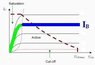# Saturation region for the BJT

Sudeesh
Hi,

It is commonly said that, the forward biased emitter-base junction and forward biased base- collector results in device saturation.

Considering a PNP transistor as an example, forward biasing the emitter- base junction results in flow of majority charges carriers(holes ) from emitter to base. Similarly, forward bias of base- collector junction results in flow of electrons from base to collector. In this case, the holes from emitter, do not reach the collector, it self. Then how can there be a larger current, when compared with the active region?

Thanks.

Saturation of a transistor is caused when the transistor is unable to draw more current from the supply regardless of the base current.

This is usually due to the size of the load resistor and the supply voltage.

For example if the supply is 12 volts and the load resistor is 1000 ohms, then the transistor's collector current cannot be more than 12 mA because this is the most current that can flow through the collector resistor. (12 volts / 1000 ohms = 12 mA).

The transistor may be able to carry many amps of collector current in another circuit, but in this circuit it will not carry more than 12 mA.

Sudeesh
For a given transistor, which one is more, saturation current or active region current?

The saturation current is about twice the active region current.

In the above example, if this was an actual amplifier, the transistor would be biased so that there was 6 volts across the transistor and 6 volts across the load resistor.

So, this would mean there was 6 mA flowing in the load resistor (and also into the collector) for use as a normal class A amplifier. (6 volts / 1000 ohms = 6 mA)

This compares with the 12 mA that would be the saturation current in this circuit.

Notice again, that the saturation current is a product of the circuit and not the transistor.

Staff Emeritus
For a given transistor, which one is more, saturation current or active region current?
For a given base current, always IC (sat) < IC (active)

see the green portion of attached characteristic

#### Attachments

•image.jpg
6.6 KB · Views: 507
Last edited:
Hi Sudeesh. Just to clarify what vk6 and Nascent have said:

- For a given supply voltage and load resistance, the maximum collector current occurs when the transistor is in saturation.

- For a given base current, the maximum collector current (excluding breakdown) occurs when the transistor is in the active region.

I hope that helps.

Kholdstare
Just to further clarify what uart has said:

- For a given supply voltage and load resistance, you draw a load line based on that and find out where the maximum current occurs. You don't look at a certain Ic-Vce curve, as Vbe can vary.

- For a given base current, you follow a single Ic-Vce curve and find out where the maxima is.